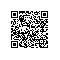# i++,i--的前置,后置++i,--i的详细解释

对i++,i--,++i,--i的易解,在此举一例子详解:main( )int x,y,z;x=y=2;z=3;y=x++-1;printf("%d\t%d\t",x,y); /*x=3,y=1,后置++,先把x赋值给y,再做运算,而x的值无论是前还是后置都加1*/y=++x-1;printf("%d\t%d\t",x,y);/*x=4,y=3,前置++,先把x加1后赋值给y,然后做运算(此行x初值是上行的终值=3)*/y=z--+1;printf("%d\t%d\t",z,y); /*z=2,y=4,同上理可知*/y=--z+1;printf("%d\t%d\t",z,y); /*z=1,y=2,同理可证*/getch();}使用钉钉扫一扫加入圈子
+ 订阅